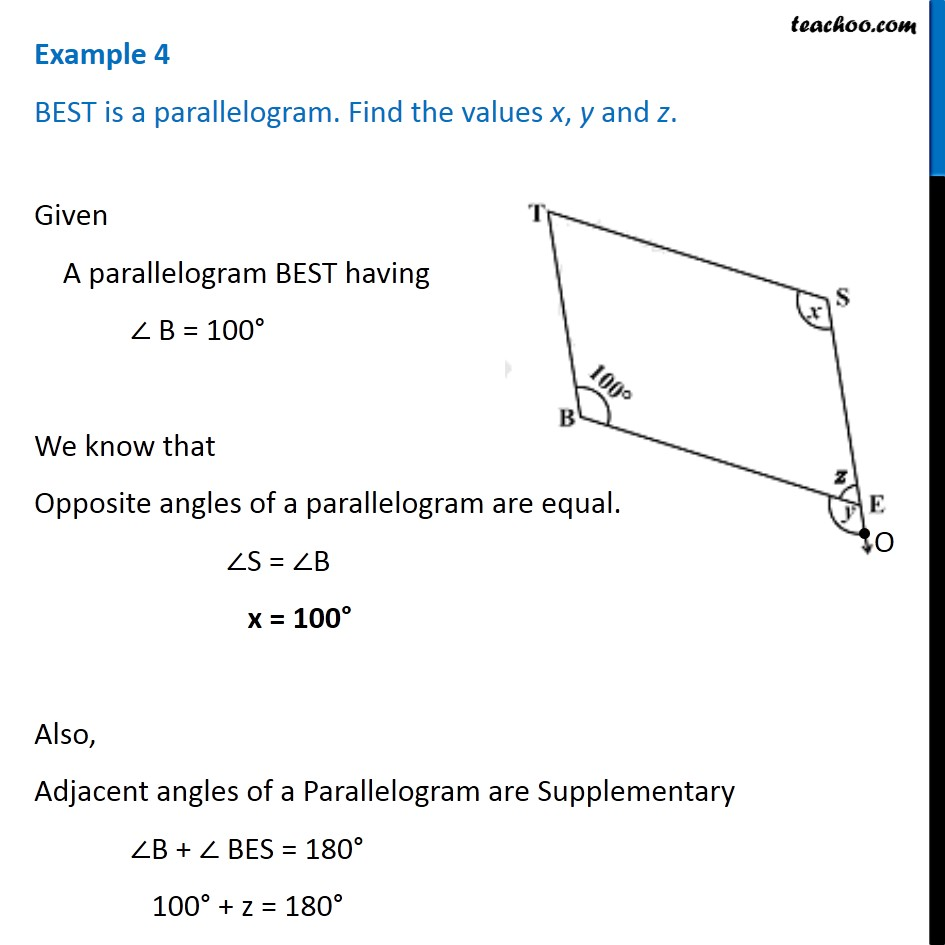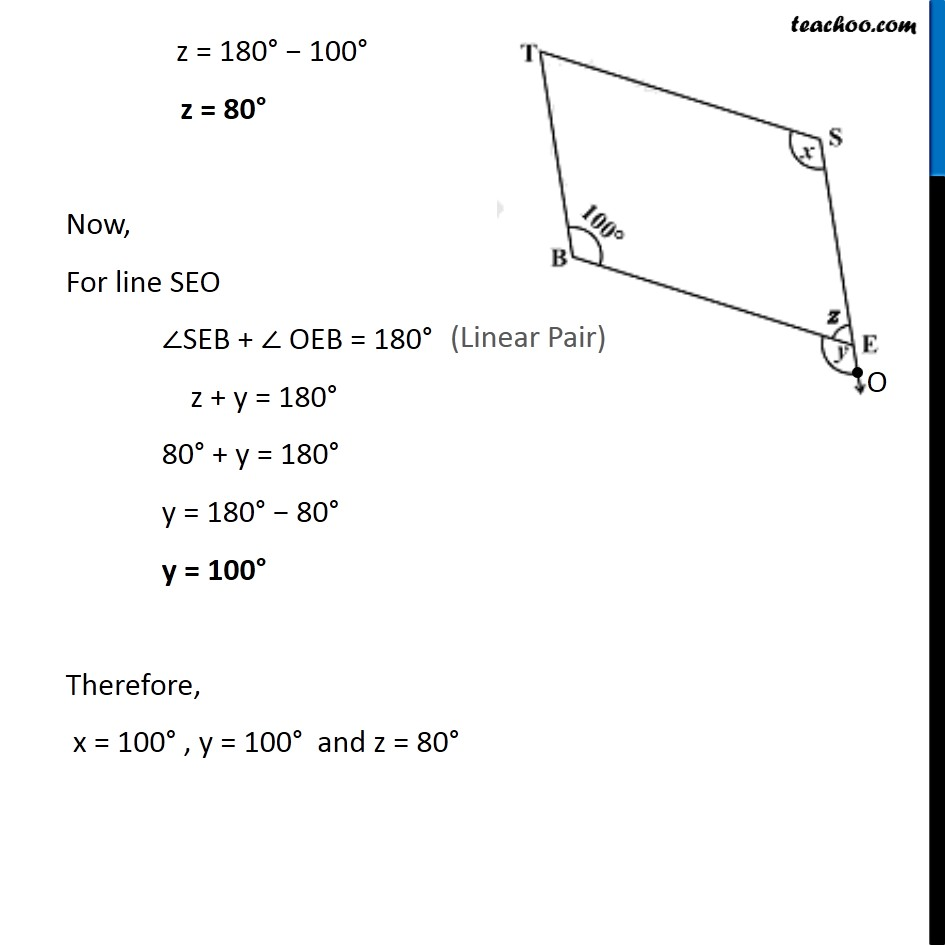Angles of Parallelogram

Chapter 3 Class 8 Understanding Quadrilaterals
Concept wiseIntroducing your new favourite teacher - Teachoo Black, at only ₹83 per month

### Transcript

Example 4 BEST is a parallelogram. Find the values x, y and z. Given A parallelogram BEST having ∠ B = 100° We know that Opposite angles of a parallelogram are equal. ∠S = ∠B x = 100° Also, Adjacent angles of a Parallelogram are Supplementary ∠B + ∠ BES = 180° 100° + z = 180° z = 180° − 100° z = 80° Now, For line SEO ∠SEB + ∠ OEB = 180° z + y = 180° 80° + y = 180° y = 180° − 80° y = 100° Therefore, x = 100° , y = 100° and z = 80°